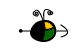pH, pOH, pKa, and pKb

The symbol "p" means "the negative of the logrithm of."  "p" is often used with very small or very large values. Calculating pH

To calculate the pH of an aqueous solution you need to know the concentration of the hydronium ion in moles per liter (molarity).  The pH is then calculated using the expression:

pH = - log [H3O+].

Example:  Find the pH of a 0.0025 M HCl solution.  The HCl is a strong acid and is 100% ionized in water.  The hydronium ion concentration is 0.0025 M.  Thus:

pH  =  - log (0.0025) = - ( - 2.60) = 2.60

Top

Calculating the Hydronium Ion Concentration from pH

The hydronium ion concentration can be found from the pH by the reverse of the mathematical operation employed to find the pH.

[H3O+] = 10-pH  or  [H3O+] = antilog (- pH)

Example:  What is the hydronium ion concentration in a solution that has a pH of 8.34?

8.34 = - log [H3O+]
- 8.34 = log [H3O+]
[H3O+] = 10-8.34 = 4.57 x 10-9 M

On a calculator, calculate 10-8.34, or "inverse" log ( - 8.34).

Calculating pOH

To calculate the pOH of a solution you need to know the concentration of the hydroxide ion in moles per liter (molarity). The pOH is then calculated using the expression:

pOH =  - log [OH-]

Example:  What is the pOH of a solution that has a hydroxide ion concentration of 4.82 x 10-5 M?

pOH = - log [4.82 x 10-5] = - ( - 4.32) = 4.32

Top

Calculating the Hydroxide Ion Concentration from pOH

The hydroxide ion concentration can be found from the pOH by the reverse mathematical operation employed to find the pOH.

[OH-] = 10-pOH   or   [OH-] = antilog ( - pOH)

Example:  What is the hydroxide ion concentration in a solution that has a pOH of 5.70?

5.70 = - log [OH-]
-5.70 = log[OH-]
[OH-] = 10-5.70 = 2.00 x 10-6 M

On a calculator calculate 10-5.70, or "inverse" log (- 5.70).

Top

Relationship Between pH and pOH

The pH and pOH of a water solution at 25oC are related by the following equation.

pH + pOH = 14

If either the pH or the pOH of a solution is known, the other can be quickly calculated.

Example:  A solution has a pOH of 11.76.  What is the pH of this solution?

pH =  14  -  pOH = 14  -  11.76 =  2.24

Top

Calculating pKa

The pKa is calculated using the expression:

pKa = - log (Ka)

where "Ka" is the equilibrium constant for the ionization of the acid.

Example:  What is the pKa of acetic acid, if Ka for acetic acid is 1.78 x 10-5?

pKa = - log (1.78 x 10-5) = - ( - 4.75) = 4.75

Top

Calculating Ka from the pKa

The Ka for an acid is calculated from the pKa by performing the reverse of the mathematical operation used to find pKa.

Ka = 10-pKa  or  Ka = antilog ( - pKa)

Example:   Calculate the value of the ionization constant for the ammonium ion, Ka, if the pKa is 9.74.

9.74 = - log (Ka)
-9.74 = log (Ka)
Ka = 10-9.74 = 1.82 x 10-10

On a calculator calculate 10-9.74, or "inverse" log ( - 9.74).

Top

Calculating pKb

The pKb  is calculated using the expression:

pKb =  - log (Kb)

where Kb is the equilibrium constant for the ionization of a base.

Example:  What is the pKb for methyl amine, if the value of Kb for methyl amine is 4.4 x 10-4?

pKb = - log (4.4 x 10-4) = - ( - 3.36) = 3.36

Top

Calculating Kb from pKb

The Kb for an acid is calculated from the pKb by performing the reverse of the mathematical operation used to find pKb.

Kb = 10-pKb  or  Kb = antilog ( - pKb)

Example:   Calculate the value of the ionization constant, Kb, for aniline if the pKb is 9.38.

9.38 = - log (Kb)
-9.38 = log (Kb)
Kb = 10-9.38 = 4.17 x 10-10

On a calculator calculate 10-9.38, or "inverse" log ( - 9.38).

Top